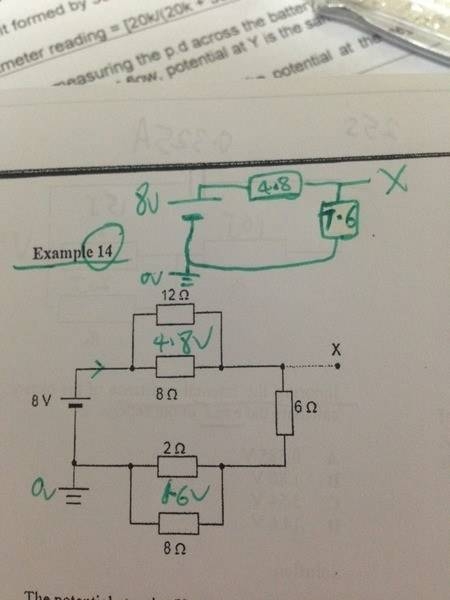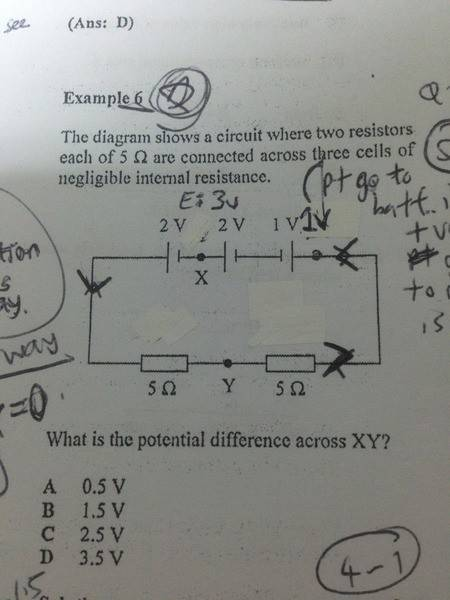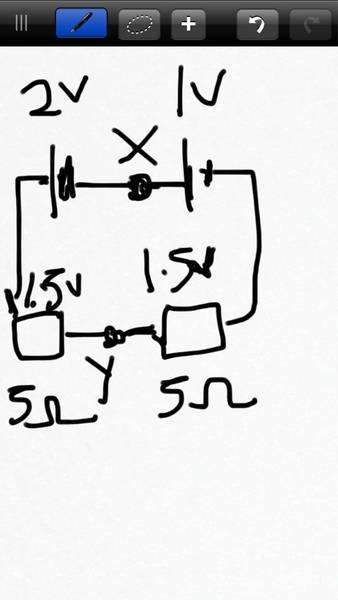# Potential at a point in a circuitWhy is the potential at X , denoted by V, not

V/8 = 4.8/(4.8+7.6) ? Why is numerator of resistance part 7.6 and not 4.8 by potential divider principle?

Homework Helper
hi vadevalor!conventionally, the current flows from the positive terminal (long line) to the negative terminal (short line)

(the electrons, of course go the other way, towards the positive!)

so the short line is at zero potential, and you have to start from the short line to get to X to find the potential at X, ie the potential difference from zero

(see eg "Electric Potential Diagrams" at http://www.physicsclassroom.com/class/circuits/u9l1c.cfm)

ie you go through the 7.6Ω, so 7.6 is in the numeratorgrzz
The values 4.8V and 1.6V shown on the circuit diagram are misleading. Should not the unit be Ω instead of V?

The equivalent circuit is a voltage supply of 8V connected to 4.8Ω in series with 7.6Ω as shown correctly in your diagram.

Hence 8V is the voltage on (4.8 + 7.6)Ω.

And the voltage on 4.8Ω can be found be using direct proportion.

Thank you all for your inputs! I realised its the direction after thinking for so long. And yea it should be ohm instead of v.

I have another question regarding potential could you guys help me? Answer is A but i dont understand the solution as there are 'conflicting battery direction' and i dont know how to startThanks!!
- curious lost soul

Homework Helper
hi vadevalor!Answer is A but i dont understand the solution as there are 'conflicting battery direction' and i dont know how to start

start by replacing the three batteries by one 3V battery …

what is the potential at Y? and where is that measured from?

and now adjust that to start from X insteadhi vadevalor!start by replacing the three batteries by one 3V battery …

what is the potential at Y? and where is that measured from?

and now adjust that to start from X insteadShould i put the 3v battery to the right or left of X and should i go from + terminal to -terminal from X to Y like current flow or electron flow?

Smiles :D

Homework Helper
hi vadevalor!Should i put the 3v battery to the right or left of X and should i go from + terminal to -terminal from X to Y like current flow or electron flow?

Smiles :D

you must always always ALWAYS use current flow not electron flow!

X is somewhere in the middle of the 3V battery, isn't it?hi vadevalor!you must always always ALWAYS use current flow not electron flow!

X is somewhere in the middle of the 3V battery, isn't it?Think i got it! Yes! So its 2-1.5= 0.5v following current direction. But when do i know to use current or electron flow direction? Above for potential divider i was told to look at electron flow

Homework Helper
yes, i like your diagram …

you've split the batteries into two: a 2V and a 2 minus 1 = 1V battery going the same way,

so it's easy to see whether to add or subtractAbove for potential divider i was told to look at electron flow

i don't understand thatit's always current flow​

what exactly did they say?hi vadevalor!conventionally, the current flows from the positive terminal (long line) to the negative terminal (short line)

(the electrons, of course go the other way, towards the positive!)

so the short line is at zero potential, and you have to start from the short line to get to X to find the potential at X, ie the potential difference from zero

(see eg "Electric Potential Diagrams" at http://www.physicsclassroom.com/class/circuits/u9l1c.cfm)

ie you go through the 7.6Ω, so 7.6 is in the numeratorIts the third paragraph from your first answer :) starting from the short line (electron flow direction)

ie we use a positive charge (ie not an electron), and so it will flow outside the battery from the long line (+ve) to the short line (-ve), and the potential will be highest at the long line, and decrease to zero at the short line